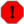## Name

ST_Length — Returns the 2d length of the geometry if it is a linestring or multilinestring. geometry are in units of spatial reference and geography are in meters (default spheroid)

## Synopsis

`float ST_Length(`geometry a_2dlinestring`)`;

`float ST_Length(`geography geog, boolean use_spheroid=true`)`;

## Description

For geometry: Returns the cartesian 2D length of the geometry if it is a linestring, multilinestring, ST_Curve, ST_MultiCurve. 0 is returned for areal geometries. For areal geometries use ST_Perimeter. Geometry: Measurements are in the units of the spatial reference system of the geometry. Geography: Units are in meters and also acts as a Perimeter function for areal geogs.

Currently for geometry this is an alias for ST_Length2D, but this may change to support higher dimensions.Changed: 2.0.0 Breaking change -- in prior versions applying this to a MULTI/POLYGON of type geography would give you the perimeter of the POLYGON/MULTIPOLYGON. In 2.0.0 this was changed to return 0 to be in line with geometry behavior. Please use ST_Perimeter if you want the perimeter of a polygonFor geography measurement defaults spheroid measurement. To use the faster less accurate sphere use ST_Length(gg,false);This method implements the OpenGIS Simple Features Implementation Specification for SQL 1.1. s2.1.5.1This method implements the SQL/MM specification. SQL-MM 3: 7.1.2, 9.3.4

Availability: 1.5.0 geography support was introduced in 1.5.

## Geometry Examples

Return length in feet for line string. Note this is in feet because 2249 is Mass State Plane Feet

```SELECT ST_Length(ST_GeomFromText('LINESTRING(743238 2967416,743238 2967450,743265 2967450,
743265.625 2967416,743238 2967416)',2249));
st_length
---------
122.630744000095

--Transforming WGS 84 linestring to Massachusetts state plane meters
SELECT ST_Length(
ST_Transform(
ST_GeomFromEWKT('SRID=4326;LINESTRING(-72.1260 42.45, -72.1240 42.45666, -72.123 42.1546)'),
26986
)
);
st_length
---------
34309.4563576191
```

## Geography Examples

Return length of WGS 84 geography line

```			-- default calculation is using a sphere rather than spheroid
SELECT ST_Length(the_geog) As length_spheroid,  ST_Length(the_geog,false) As length_sphere
FROM (SELECT ST_GeographyFromText(
'SRID=4326;LINESTRING(-72.1260 42.45, -72.1240 42.45666, -72.123 42.1546)') As the_geog)
As foo;
length_spheroid  |  length_sphere
------------------+------------------
34310.5703627305 | 34346.2060960742
(1 row)
```